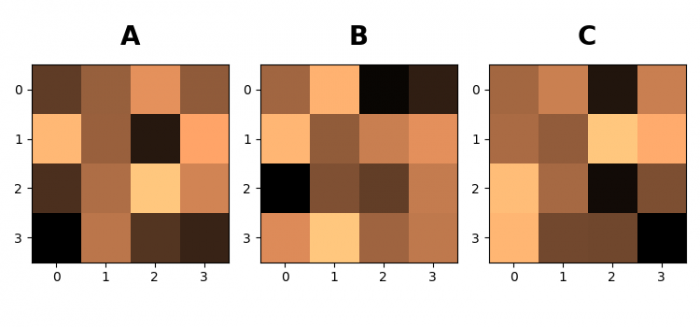# Annotate Subplots in a Figure with A, B, C using Matplotlib

To annotate subplots in a figure with A, B and C using matplotlib, we can take the following steps

• Set the figure size and adjust the padding between and around the subplots.
• Create a figure and a set of subplots, with nrows=1 and ncols=3.
• Make a 1D iterator over an array.
• Iterate each axes and display data as an image.
• In the loop itself, place text A, B and C.
• To display the figure, use show() method.

## Example

import numpy as np
from matplotlib import pyplot as plt
import string
plt.rcParams["figure.figsize"] = [7.50, 3.50]
plt.rcParams["figure.autolayout"] = True
fig, axs = plt.subplots(1, 3)
axs = axs.flat
for index, ax in enumerate(axs):
ax.imshow(np.random.randn(4, 4), interpolation='nearest',
cmap="copper")
ax.text(0.45, 1.1, string.ascii_uppercase[index],
transform=ax.transAxes,
size=20, weight='bold')
plt.show()

## Output